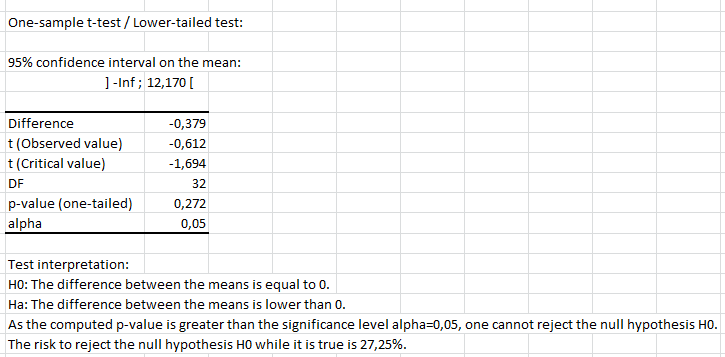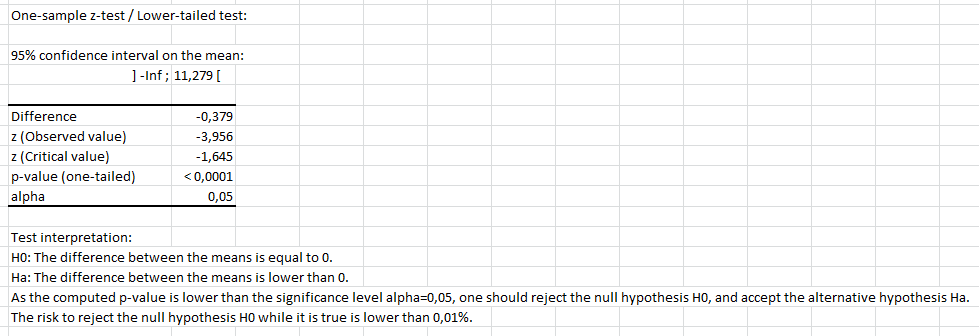# One sample t-test or z-test in Excel tutorial

2018-06-22

This tutorial will help you test the difference between an observed mean and a theoretical one, using the one sample t-test and z-tests, in Excel with XLSTAT.
Not sure this is the statistical test you are looking for? Check out this guide.

## Dataset to perform a mean comparison test - either a Student's t-test or a z-test

An Excel sheet with both the data and the results can be downloaded by clicking on the button below:

The data correspond to the mathematic grade average for the first semester of a class of 33 students.

## Goal 1 of this tutorial

We want to compare the results of those students with the county average which is 11.5. We don’t have the information about the variance of this statistic so we cannot run a z-test. We will then run a t-test.

The class average is 11.121. Descriptive statistics on the class performance can be computed using the function Descriptive Statistics.

We can use a t-test to determine if the difference between the average of the class and the average of all students in the county are similar or different with a risk of 5%.

## Setting up a Student's t-test for comparing the mean of a sample to a value

Go over to the menu Parametric tests and select the option One-sample t-test and z-test.In the dialog box, select the data. Press the Range selector and select the column B that contains the “Math average”. The data format is One sample per column. Here we have only one column corresponding to one sample to test. If the data were spanned on several columns for the same sample we could use the other option.

The results will be displayed in a new sheet as we keep the default option Sheet.

The name of the column was included in our data selection so we tick the option Column labels.

Finally, you have to decide if you want to run a t-test or a z-test. As explained above we don’t have the information about the standard deviation of the population so we have to run a Student’s t test.We can move on to the tab Options to specify our analysis. The first thing to select is the alternative hypothesis. We know that the average of the class is inferior to the general average so we can select the option Mean 1 < Theoretical mean to run a one-sided test which is more accurate than a two-sided test.

Then we enter 11.5 in the Theoretical mean field. The default significance level is 5%. We will use this value.In the Missing data tab, we can choose any option as we do not have missing data in this dataset. For the last section Outputs, we select the only available option Descriptive statistics.When everything is set, press OK.

## Results of the Student's t-test to compare the mean of a sample to a value

The results of the t-test appear in a new sheet. The following table shows that we cannot reject the null hypothesis Ho so we may suggest that the average class performance is as good as the average performance of the country.## Goal 2 of this tutorial

To go further we can now use the standard deviation for the math grades for the county. Let’s use 0.55. So the variance is 0.3025.

## Setting up a z-test for the comparison of the mean of a sample to a value

In the first tab General, this time instead of the Student’s t-test option choose the option z-test.In the Options tab, you need to set the variance for the z-test. Opt for the option User defined and set the value to 0.3025.Click OK.

## Results and interpretation of a z-test for the comparison of the mean of a sample to a value

In the results page you now have that the hypothesis H0 should be rejected and that the students performed significantly worse than the students of the county.The following video shows how to run a Student's t-test and a z-test in XLSTAT.

#### Contact our technical support team: support@xlstat.com

https://cdn.desk.com/
false
desk